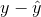# AP Statistics : How to create residual plots

## Example Questions

### Example Question #1 : How To Create Residual Plots

On a residual plot, the-axis displays the __________ and the-axis displays __________.

Possible Answers:

independent variable; the dependent variable

independent variable;residuals; the independent variable

dependent variable; residualsresiduals; theresiduals

Correct answer:

independent variable;Explanation:

A residual plot shows the difference between the actual and expected value, or residual. This goes on the y-axis. The plot shows these residuals in relation to the independent variable.

### All AP Statistics Resources